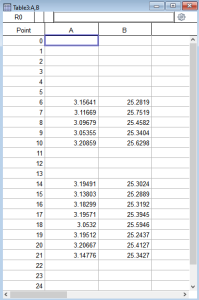# How to get the mean and std. of bits (fragments) of data?Hi, I have long waves with uneven bits of data. I would like to get the mean and std. of each bit, how could I do this? I guess it should be a loop that goes from one bit to the next, but I don’t know how to do this.I'm not super certain of what you're asking for... below is a snippet that will return a 2D free wave with the average and standard deviation of each contiguous chunk of data only; so, e.g.if there are two contiguous chunks like shown in your image, then the result will be a 2x2 matrix. There may be more efficient ways to do this, and you may not want just the values if you have timeseries data for example.

//___________________________________________________________________________________________________
// Get average and standard deviation of each chunk of data.
Function/WAVE StatsNonNaNs(Wave inWv)

int i,k,q,n=numpnts(inWv)
Make /FREE /N=(0,2) outWv = NaN

do
if (numtype(inWv[i]) == 0)
k=i
do
k++
while ( (k < i) && (k < n) )
q=k-1
if (numtype(inWv[k]) == 0)
do
k++
if (k == n)
break
endif
while ((numtype(inWv[k]) == 0) && (k < n))
k--
if ((k-q)  >= 1)
InsertPoints /M=0 Inf, 1, outWv
WaveStats/Q/R=[q, k]/M=2 inWv
outWv[Inf] = V_avg
outWv[Inf] = V_sdev
endif
i=k
else
i=k
endif
else
i++
endif
while (i < n-1)

return outWv
end
//___________________________________________________________________________________________________